# Trigonometry

### Pythagoras Theoram:

For all the right angled triangles “the square on the hypotenuse (c2) is equal to the sum of all the squares on the other two sides (a2 + b2 ).

#### 🚚 Delivering all over PAKISTAN 🇵🇰 & ✈️Internationally 🌐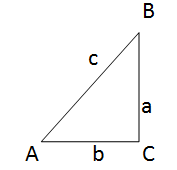c2 = a2 + b2

### Trigonometrical Ratios of Acute Angle:

All angles in a right angle triangle are acute angles. (between 0 to 90 degrees).

### Sine: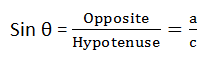### Cosine: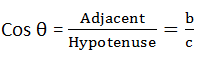### Tangent: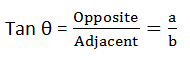Tip to remember this SOH CAH TOA.

Example:

Q: Find the unknown values of :Sin 30 = Opposite / Hypotenuse

Sin 30 = x / 13

X = 6.5 Ans

Y2 + X2 = 102

Y2 = 102 –  6.52

Y = 7.60 (Ans)

### Trigonometrical Ratios of Obtuse Angles:

The trigonometric ratios of an obtuse angle (between 90 and 180 degree) can be expressed in terms of the adjacent acute angle that lies in the same straight line.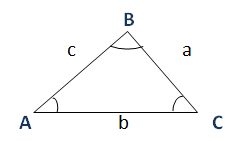### Area of Triangle:

For a known right angled triangle with any two sides given and the area.

Area = ½ ab sin C

Area = ½ bc sin A

Area = ½ ac sin B

### Sine Rule:It can be used when:

• Two angles and one side given.
• Two sides and one non-included angle given.

### Cosine Rule:

a2= b2 = c2 – (2bc cos A)

b2= a2 = c2 – (2ac cos B)

c2= a2 = b2 – (2ab cos C)

It can be used when:

• Three sides are given.
• Two sides and one angle is given.

### 😃 Relax your Mind From Studying and WATCH this Beautiful Painting! 👇